Maths-
General
Easy

Question

# The perimeter of the triangle is 44cm. if its sides are in the ratio 9:7:6. Find its area.Hint:

## The correct answer is: 8√110 cm2

### It is given that  perimeter of the triangle = 44cmi.e. Sum of all sides = 44 cm9x + 7x + 6x = 44  ⇒ 22x = 44  ⇒ x = 2So, a = 18 cm , b = 14 cm and c = 12 cmUsing Heron’s formula,Area of triangle =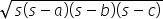where s =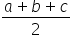s =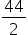= 22Area of triangle =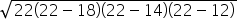=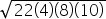=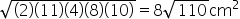#### With Turito Foundation.#### Get an Expert Advice From Turito.